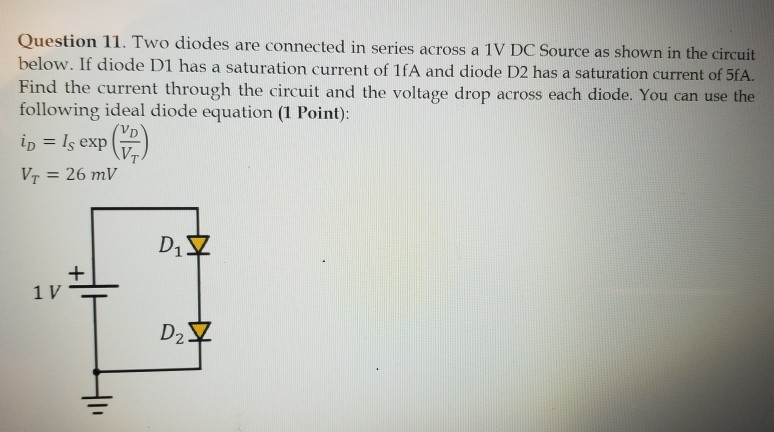Home / Answered Questions / Other / question-11-two-diodes-are-connected-in-series-across-a-1v-dc-source-as-shown-in-the-circuit-below-i-aw977

# (Solved): Question 11. Two Diodes Are Connected In Series Across A 1V DC Source As Shown In The Circuit Below....Question 11. Two diodes are connected in series across a 1V DC Source as shown in the circuit below. If diode D1 has a saturation current of 1fA and diode D2 has a saturation current of 5fA. Find the current through the circuit and the voltage drop across each diode. You can use the following ideal diode equation (1 Point): id = 1, exp) Vj = 26 mV DV 11t1 Dz.

We have an Answer from Expert